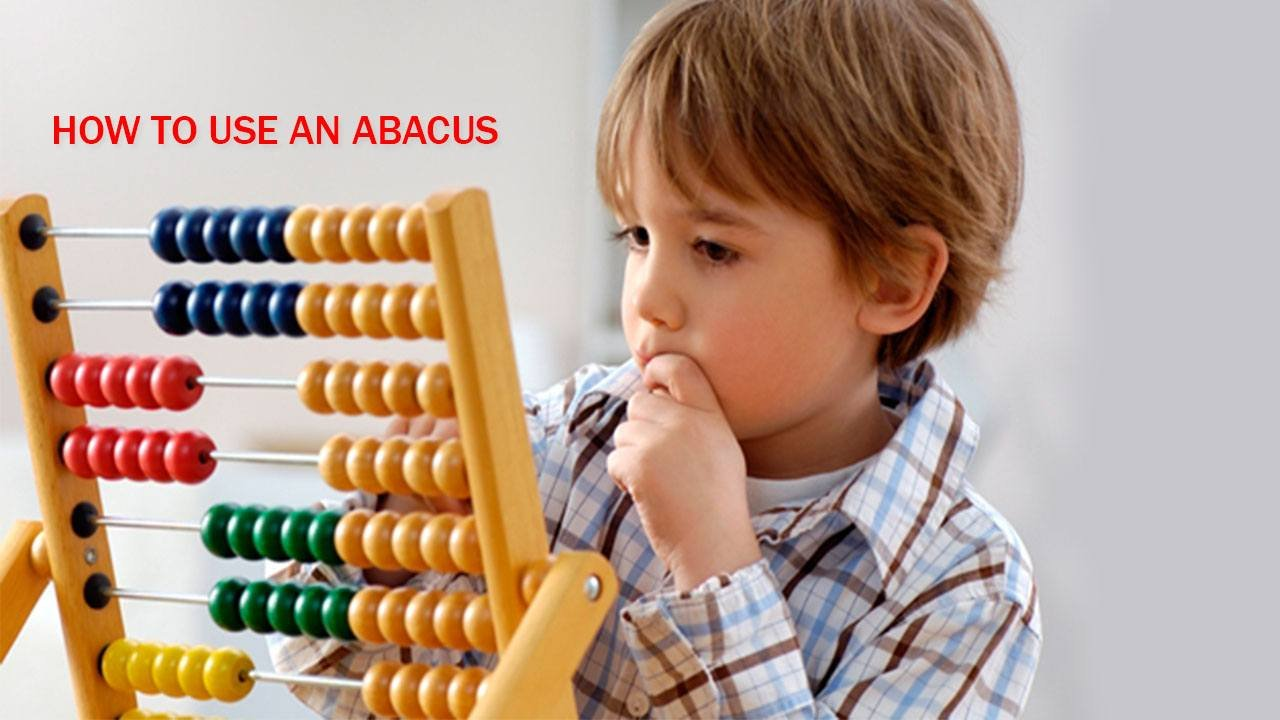Call: +91-9717294447# Live 1:1 Online Abacus Classes

Kids learn the fundamentals of Abacus that helps them think analytically and have better reasoning abilities. Analytical and reasoning skills are essential because it helps them to solve problems and look for solutions.

All lessons are taught Live 1:1 Online in the convenience of your home by Top 99.9th percentile of certified tutors.#### About the training program:

Children from 4 to 15 years old can learn ABACUS. You don’t need to use a calculator or computer to learn Abacus. Abacus is the best way for all children to learn arithmetic without a calculator, pencil and paper.

WE DIVIDED ABACUS PROGRAM INTO THREE CATEGORIES-### Abacus (Basic)

Here is a list of all the Abacus Level skills that Basic Level students (From age 4-6 years old) have learned! These skills will teach by our expert Tutors.

Addition and subtraction without Compliments (16 sessions 2 months)

• Single digit, 5 rows
• Single digit, 6 rows
• Single digit, 7 rows
• Single digit, 8 rows
• Single digit, 9 rows

Addition & Subtraction with Compliments (16 sessions 2 months)

• Single digit, 5 rows, Compliments of 5
• Single digit, 5 rows, Compliments of 10
• Single digit, 6 rows, Mixed Compliments
• Single digit, 7 rows, Mixed Compliments
• Single digit, 8 rows, Mixed Compliments

### Abacus (Intermediate)

Here is a list of all the Abacus Level skills that Intermediate Level students (From age 5-10 years old) have learned! These skills will teach by our expert Tutors.

Addition & Subtraction, Multiple Digits(16 sessions 2 months)

• Single digit, 12 rows
• Double digit, 5 rows
• Double digit, 7 rows
• Triple digit, 3 rows
• Triple digit, 4 rows

Addition, Subtraction & Multiplication(16 sessions 2 months)

• Double digit, 7 row, addition/subtraction
• Triple digit, 4 rows, addition/subtraction
• Triple digits, 7 row, addition/subtraction
• Single digit x Double digit Multiplication
• Single digit x Triple digit Multiplication
• Double digit x Double digit

Addition, Subtraction and Multiplication(16 sessions 2 months)

• Three digits, 4 rows
• Four digits, 4 rows
• Double digit x Double digit Multiplication
• Triple digit x Double digit Multiplication
• Triple digit x Triple digit Multiplication

Here is a list of all the Abacus Level skills that Advance Level students (From age 9-15 years old) have learned! These skills will teach by our expert Tutors.

Multiplication and Division(16 sessions 2 months)

• Double digits x Double digit Multiplication
• Triple digits x Double digit Multiplication
• Two digit no. division by single digit
• Triple digit no. division by single digit
• Four digit no. division by single digit

Multiplication, Division & Decimal addition/subtraction (16 sessions 2 months)

• Triple digit no. division by Double digit
• Four digits no. division by Double digit
• Four digit no. division by Triple digit
• Decimal addition & subtraction, 4 rows

Decimal Multiplication, Division, L CM, GCD  (16 sessions 2 months)

• Decimal Multiplication, ALL TYPES
• Decimal Division, ALL TYPES
• LCM
• GCD